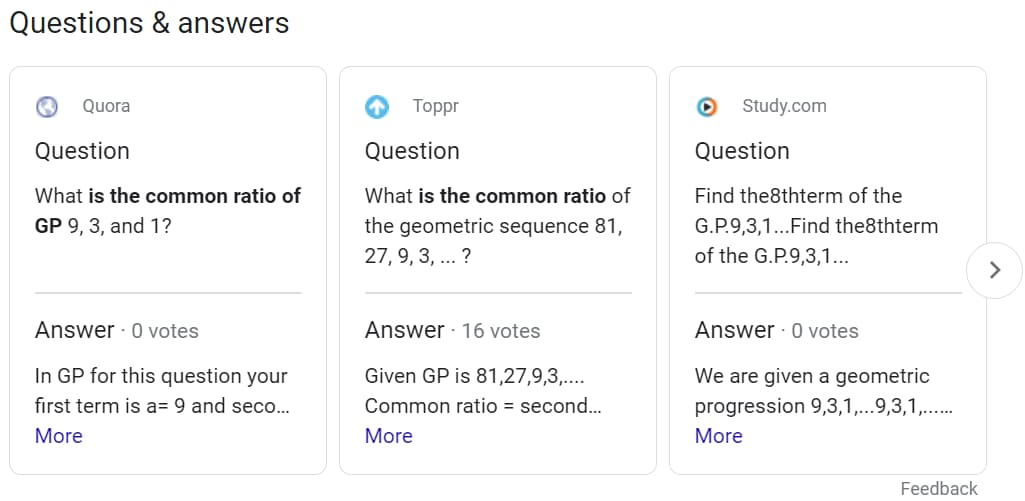The Google Questions And Answers API allows a user to scrape questions and answers from a regular Google Search page. SerpApi is able to make sense of this information and extract `link`, `source`, `question`, `answer`, and `votes`.

## API Examples

### Results for: `Common ratio of gp 931`JSON Example

``````{
...
{
"source": "Quora",
"question": "What is the common ratio of GP 9, 3, and 1?",
"answer": "In GP for this question your first term is a= 9 and second term is at= 3 and third term is ar^2 to find the ratio second term is divided by first term and third term is divided by second term and so on 3/9= 1/3 1/3=1/3 therefore its common ratio is 1/3",
},
{
"source": "Toppr",
"question": "What is the common ratio of the geometric sequence 81, 27, 9, 3, ... ?",
"answer": "Given GP is 81,27,9,3,.... Common ratio = second numberfirst number = 2781 = 13 So, the common ratio is 13 .",